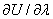From: mashaojie163 (mashao_jie_at_163.com)
Date: Mon Dec 27 2004 - 01:29:36 CST

Dear Sir:
I am obscure for Free Energy Calculations (Conformational forcing / Potential of mean force). As we have know:
In MCTI method. It accumulates at several values of lambda, and from these averages estimates
the integral,

With this method,the precision of each can be estimated from the fluctuations of the time series of .

In my freeEnergyConfig, I define as below:

urestraint {
pos pmf (INI, 2) kf=20 low=(-2.2 5.8 1.8) hi=(-2.2 6.8 1.8)
}
mcti {
equiltime = 10 ps
accumtime = 10 ps
numsteps = 10
print = 0.1 ps
}
In my opinion, "numsteps = 10" means lambda values vareis in the range (0.1 0.2 0.3 ... 0.9 1.0). For each lambda, lambda is invariable, was not calculated in equiltime(first 10 ps) while it was calculated every timestep(2 fs) in accumtime (later 10 ps). was outputed every 0.1 ps. Its value is delta-G in log file. I think it is necessary to output (the statistic mean value of ) for each lamda, because single delta-G is no sense and it does not correspond to a lambda (In Slow growth method,
every single delta-G correspond to a lambda). So I think if use MCTI, output for each lambda is necessary.

Is right what I thought? Pls tell me the errors in my opinion.. Can you tell me which source files are about FreeEnergy calcalation.? Are there some program user guide?This archive was generated by hypermail 2.1.6 : Wed Feb 29 2012 - 15:39:04 CST